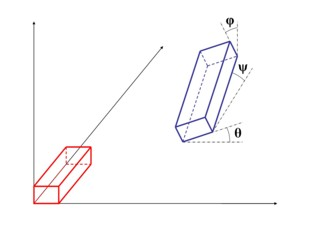# Definition of two conductors at an angle

## Identification strategy

To identify, from a geometrical point of view, two volume conductors positioned at an angle between them, the strategy adopted here relies on the angles of:

• sharp bend
• pitching
• rolling

These three angles are identified in relationship with the direction of the current that passes through the two conductors.

The diagram below illustrates this definition.

## Diagram• ψ: sharp bend angle
• φ: pitching angle
• θ: rolling angle